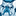# QlikView App Dev

Discussion Board for collaboration related to QlikView App Development.

Announcements
Support Case Portal has moved to Qlik Community! Read the FAQs to start exploring Support resources.
cancel
Showing results for
Did you mean:Contributor II

## Rounding off a number- how to actually change it?

I have two fields of numbers I am comparing: one which is calculated (and therefore it has many digits potentially after the decimal), and one which already only has 2 decimal places.

For example, assume my script is as follows:

Sales

Percentage

Sales * Percentage as [Number 1]

[Number 2]

The result for Number 1 might in example be 345.98765

Assume Number 2 for this record is 345.99

I then have a flag calculated along the lines of: if( [Number 1]=[Number 2], 'OK', 'Not OK') as NumberFlag

Currently, the fact pattern above would result in a 'Not OK' for this record because Qlik is comparing the full result of Number 1 to Number 2, and determining that Number 2 is greater. But what I want is for Qlik to first round Number 1 to 345.99 and then compare that rounded value to Number 2, which would return an 'OK' result.

I've tried using the rounding function and the num function as follows:

round((Sales * Percentage),0.01) as [Number 1]

num((Sales * Percentage),'##.##') as [Number 1]

But neither of these seems to actually change the underlying amount. Number 1 will show up in a table as 345.99, but the NumberFlag will be 'Not OK'.

What am I missing here?

2 RepliesMVP

I think this blog will clearly explain the issue to you -> Rounding ErrorsMVP

chk dis

or else numb tab u cn select  Fixed  value to  "2"

Rounding ErrorsCommunity Browser